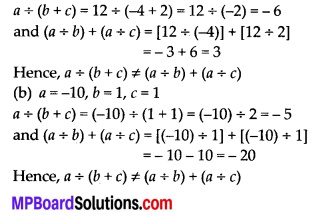# MP Board Class 7th Maths Solutions Chapter 1 Integers Ex 1.4

## MP Board Class 7th Maths Solutions Chapter 1 Integers Ex 1.4

Question 1.
Evaluate each of the following:
(a) (-30) ÷ 10
(b) 50 ÷ (-5)
(c) (-36) ÷ (-9)
(d) (- 49) ÷ (49)
(e) 13 – 5 ÷ [(-2) + 1 ]
(f) 0 – 5 ÷ (-12)
(g) (-31) ÷ 5 – [(-30) + (-1)]
(h) [(-36) ÷ 12] ÷ 3
(i) [(- 6) + 5] ÷ [(-2) + 1 ]
Solution:
(a) (-30) ÷ 10 = – 3
(b) 50 ÷ (-5) = -10
(c) (-36) ÷ (-9) = 4
(d) (- 49) ÷ (49) = – 1
(e) 13 ÷ [(-2) + 1] = 13 ÷ [-1] = -13
(f) 0 + (-12) = 0
(g) (-31) + [(-30) + (-1)] = (-31) ÷ [-31] = 1
(h) [(-36) – 12] ÷ 3 = [-3] ÷ 3 = – 1
(i) [(-6) + 5] ÷ [(-2) + 1] = (-1) ÷ (-1) = 1Question 2.
Verify that a ÷ (b ÷ c) ≠ (a ÷ b) + (a ÷ c) for each of the following values of o, b and c.
(a) a = 12, b = -4, c = 2
(b) a = -10, b = 1, c = 1
Solution:
(a) a = 12, b = -4, c = 2Question 3.
Fill in the blanks:
(a) 369 ÷ ___ = 369
(b) (-75) ÷ ____ = -1
(c) (-206) ÷ ___ = 1
(d) -87 ÷ ___ = 87
(e) ___ ÷ 1 = – 87
(f) ___ ÷ 48 = -1
(g) 20 ÷ ___ = -2
(h) ___ ÷ (4) = -3
Solution:
(a) 369 ÷ 1 =369
(b) (-75) ÷ 75 = -1
(c) (-206) ÷ (-206) = 1
(d) -87 ÷ (-1) = 87
(e) (-87) ÷ 1 = -87
(f) (-48) ÷ 48 = -1
(g) 20 ÷ (-10) = -2
(h) (-12) ÷ (4) = -3Question 4.
Write five pairs of integers (a, b) such that a + b = -3. One such pair is (6, -2) because 6 ÷ (-2) = (-3).
Solution:
(i) (-6, 2) because – 6 ÷ 2 = -3
(ii) (12, -4) because 12 ÷ (- 4) = -3
(iii) (-12, 4) because (-12) ÷ 4 = -3
(iv) (-15, 5) because (-15) ÷ 5 = -3
(v) (15, -5) because 15 ÷ (-5) = -3

Question 5.
The temperature at 12 noon was 10°C above zero. If it decreases at the rate of 2°C per hour until midnight, at what time would the temperature be 8°C below zero? What would be the temperature at mid-night?
Solution:
Initial temperature at 12 noon = 10°C
Change in temperature per hour = – 2°C
Temperature at
1:00 p.m. = 10°C + (-2°C) = 8°C
2:00 p.m. = 8°C + (-2°C) = 6°C
3:00 p.m. = 6°C + (-2°C) = 4°C
4:00 p.m. = 4°C + (-2°C) = 2°C
5:00 p.m. = 2°C + (-2°C) = 0°C
6:00 p.m. = 0°C + (-2°C) = -2°C
7:00 p.m. = -2°C + (-2°C) = -4°C
8:00 p.m. = -4°C + (-2°C) = -6°C
9:00 p.m. = -6°C + (-2°C) = -8°C
Hence, at 9:00 p.m. the temperature will be 8°C below zero.
Now, temperature at 10:00 p.m. = -8°C + (-2°C) = -10°C 11:00 p.m. = – 10°C + (-2°C) = -12°C 12:00 a.m. = -12°C + (-2°C) = – 14°C
Hence, at mid-night the temperature will be -14°C.Question 6.
In a class test (+ 3) marks are given for every correct answer and (-2) marks are given for every incorrect answer and no marks for not attempting any question,
(i) Radhika scored 20 marks. If she has got 12 correct answers, how many questions has she attempted incorrectly?
(ii) Mohini scores -5 marks in this test, though she has got 7 correct answers. How many questions has she attempted incorrectly?
Solution:
Marks obtained for 1 correct answer = +3
Marks obtained for 1 incorrect answer = – 2
(i) Marks scored by Radhika = 20
Marks obtained for 12 correct answers
= 12 × 3 = 36
Marks obtained for incorrect answers = Total score – Marks obtained for correct answers = 20 – 36 = – 16
Marks obtained for 1 incorrect answer = – 2
Thus, number of incorrect answers = (-16) + (-2) = 8
Therefore, she attempted 8 questions incorrectly.

(ii) Marks scored by Mohini = – 5
Marks obtained for 7 correct answers = 7 × 3 = 21
Marks obtained for incorrect answers = Total score – Marks obtained for correct answers = -5 – 21 = -26
Marks obtained for 1 incorrect answer = – 2
Thus, number of incorrect answers = (-26) ÷ (-2) = 13
Therefore, she attempted 13 questions incorrectly.Question 7.
An elevator descends into a mine shaft at the rate of 6 m/min. If the descent starts from 10m above the ground level, how long will it take to reach – 350 m.
Solution:
Distance descended is denoted by a negative integer.
Initial height = + 10 m
Final depth = – 350 m
Total distance to be descended by the elevator = (-350) – (+10) = – 360 m
Time taken by the elevator to descend (-6) m = 1 min
Thus, time taken by the elevator to descend (- 360) m = (-360) ÷ (-6)
= 60 minutes = 1 hour